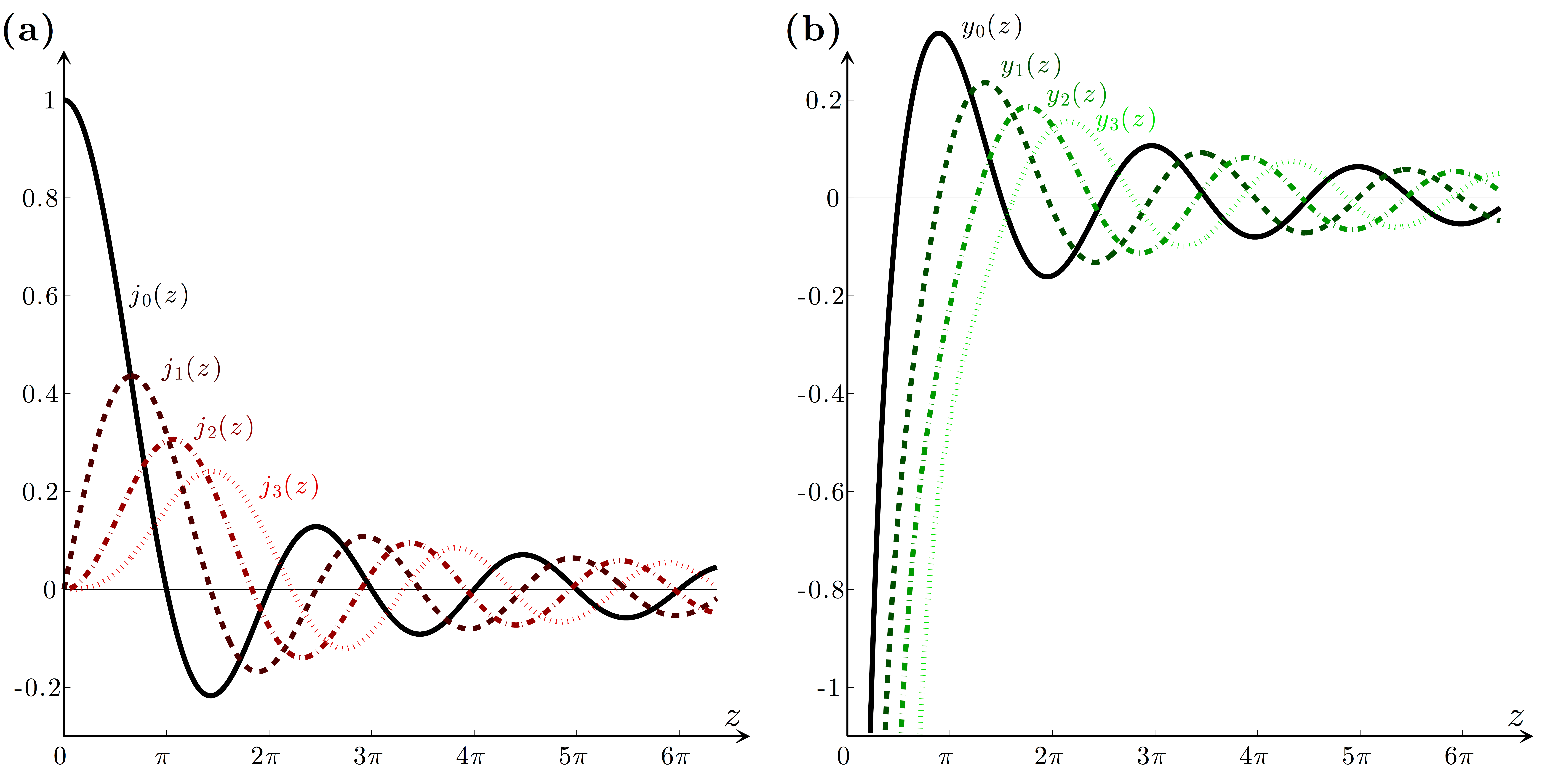# Figure 5.3 — Spherical Bessel functionsFigure 5.3 — Spherical Bessel functions. The first few (a) spherical Bessel functions jl(z) [Eq. (5.50)] and (b) spherical Neumann functions yl(z) [Eq. (5.51)]. When z is real, jl(z) and yl(z) are respectively equal to the real and imaginary part of the Hankel function hl(z) [Eq. (5.52)].